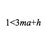# Help! Horizontal Asymptote!

Once again, I'm coming to Brilliant with another question. This time, it's on the limits of probabilities.

I was investigating this problem:

You are playing a computer game with $n-1$ of your friends (a total of $n$ people), and each of you get a chest with a $\frac{1}{n}$ chance of giving you a prize. What is the probability that none of you get a prize?

I realized that each party member has a $\frac{n-1}{n}$ chance of not getting a prize. This approaches 100% as you have more and more party members. The party probability is easy to find once you find the individual probability. Since the probability for each party member is $\frac{n-1}{n}$, you just need to raise it to the $n^{\text{th}}$ power, since there are $n$ people in the party.

Then, I got curious about the infinite limit of the function: $\displaystyle\lim_{x\rightarrow \infty} f(x)=\left(\frac{x-1}{x}\right)^x$ After I graphed it, I realized that there is a horizontal asymptote, and it cannot be calculated through traditional methods. Could someone explain how to get the solution (not just through WolframAlpha...)? I know it is approximately $y=.368$ through direct observation.

It is actually very interesting that, if there is an arbitrarily large amount of people, the probability that no one will get a prize is more than a third! Talk about bad odds!Note by Blan Morrison
3 years ago

This discussion board is a place to discuss our Daily Challenges and the math and science related to those challenges. Explanations are more than just a solution — they should explain the steps and thinking strategies that you used to obtain the solution. Comments should further the discussion of math and science.

When posting on Brilliant:

• Use the emojis to react to an explanation, whether you're congratulating a job well done , or just really confused .
• Ask specific questions about the challenge or the steps in somebody's explanation. Well-posed questions can add a lot to the discussion, but posting "I don't understand!" doesn't help anyone.
• Try to contribute something new to the discussion, whether it is an extension, generalization or other idea related to the challenge.

MarkdownAppears as
*italics* or _italics_ italics
**bold** or __bold__ bold
- bulleted- list
• bulleted
• list
1. numbered2. list
1. numbered
2. list
Note: you must add a full line of space before and after lists for them to show up correctly
paragraph 1paragraph 2

paragraph 1

paragraph 2

[example link](https://brilliant.org)example link
> This is a quote
This is a quote
    # I indented these lines
# 4 spaces, and now they show
# up as a code block.

print "hello world"
# I indented these lines
# 4 spaces, and now they show
# up as a code block.

print "hello world"
MathAppears as
Remember to wrap math in $$ ... $$ or $ ... $ to ensure proper formatting.
2 \times 3 $2 \times 3$
2^{34} $2^{34}$
a_{i-1} $a_{i-1}$
\frac{2}{3} $\frac{2}{3}$
\sqrt{2} $\sqrt{2}$
\sum_{i=1}^3 $\sum_{i=1}^3$
\sin \theta $\sin \theta$
\boxed{123} $\boxed{123}$

Sort by:

Well, since the probability of a person getting a prize depends on the number of people themselves, the result is not so surprising.

How did you stumble upon this problem?

Actually, this problem is based off of an actual scenario that I was involved in. I just got curious and decided to generalize it.

- 3 years ago

Did you realise that the number you produced is actually $\frac{1}{e}$? You have a real scenario? That is interesting. Can you tell me about it?

Oh my goodness; you're correct! That's crazy. Did you actually work it out, or were you just messing around? If you did calculate it, how so?

Scenario: I play this mobile game called Clash Royale. They recently added a feature where everyone in your group, called a clan, gets a chest after winning in an event. The winning chest has a 1 in 10 chance of giving you a special prize. Now, my clan has 30 members, so I knew that I should expect 3 people in my clan to get the prize. Then, I started getting curious about the probability of no one getting a prize. You can infer what happened from there: I calculated it, decided to generalize for a clan of $n$ members with a 1 in $n$ chance of getting the prize, and then I got here.

- 3 years ago

Well, it is an well known fact that $e^x = \lim_{n \to \infty} ( 1 + \frac{x}{n})^n$

I am not sure I am following. Why does the chance of someone getting a prize in the clan reduce when there are more people?

Ah, I see. Replacing $x$ with -1 gives you that same function, which makes the limit equal to $e^{-1}$. What is the name of this famous limit? I've never seen it.

In the original scenario, the probability of getting the prize was independent of the number of members in the clan. I modified that scenario, giving us the one mentioned in the note.

- 3 years ago

I don't really know what it is called. I learnt it in high school.

Cool. Maybe you can post this as a problem?

Here it is! Thanks for the help!

- 3 years ago